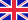•# Queueing theory

Autor: Source: Wikipedia

Source: Wikipedia. Pages: 32. Chapters: Arrival theorem, Balance equation, BCMP network, Birth-death process, Bulk queue, Burke's theorem, Buzen's algorithm, Cumulative flow diagram, Ehrenfest model, Erlang (unit), Fluid queue, Fork-join queue, G-network,... Viac o knihe

Na objednávku, dodanie 2-4 týždne

14.37 €

bežná cena: 16.90 €

## O knihe

Source: Wikipedia. Pages: 32. Chapters: Arrival theorem, Balance equation, BCMP network, Birth-death process, Bulk queue, Burke's theorem, Buzen's algorithm, Cumulative flow diagram, Ehrenfest model, Erlang (unit), Fluid queue, Fork-join queue, G-network, Gordon-Newell theorem, Jackson network, Kendall's notation, Kingman's formula, Lindley equation, Little's law, M/G/1 queue, M/M/1 queue, M/M/c queue, Markovian arrival processes, Mean value analysis, Offered load, Palm calculus, Palm-Khintchine theorem, Pollaczek-Khinchine formula, Product form solution, Punctuality, Quasi-birth-death process, Quasireversibility, Queueing model, Queuing delay, Teletraffic engineering, Traffic equations, Traffic generation model, Uniformization (probability theory). Excerpt: In queueing theory, a discipline within the mathematical theory of probability a Jackson network (sometimes Jacksonian network) is a class of queueing network where the equilibrium distribution is particularly simple to compute as the network has a product-form solution. It was the first significant development in the theory of networks of queues, and generalising and applying the ideas of the theorem to search for similar product-form solutions in other networks has been the subject of much research, including ideas used in the development of the Internet. The networks were first identified by James R. Jackson and his paper was re-printed in the journal Management Science's 'Ten Most Influential Titles of Management Sciences First Fifty Years.' Jackson was inspired by the work of Burke and Reich, though Walrand notes "product-form results ... a much less immediate result of the output theorem than Jackson himself appeared to believe in his fundamental paper". An earlier product-form solution was found by R. R. P. Jackson for tandem queues (a finite chain of queues where each customer must visit each queue in order) and cyclic networks (a loop of queues where each customer must visit each queue in order). A Jackson network consists of a number of nodes, where each node represents a queue in which the service rate can be both node-dependent and state-dependent. Jobs travel among the nodes following a fixed routing matrix. All jobs at each node belong to a single "class" and jobs follow the same service-time distribution and the same routing mechanism. Consequently, there is no notion of priority in serving the jobs: all jobs at each node are served on a first-come, first-served basis. Jackson networks where a finite population of jobs travel around a closed network also have a product-form solution described by the Gordon-Newell theorem. In an open network, jobs arrive from outside following a Poisson process with rate . Each arrival is independently routed to node j w

• Vydavateľstvo: Books LLC, Reference Series
• Rok vydania: 2013
• Formát: Paperback
• Rozmer: 246 x 189 mm
• Jazyk: Anglický jazyk
• ISBN: 9781157414209

Generuje redakčný systém BUXUS CMS spoločnosti ui42.